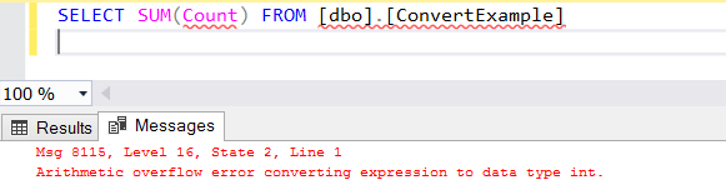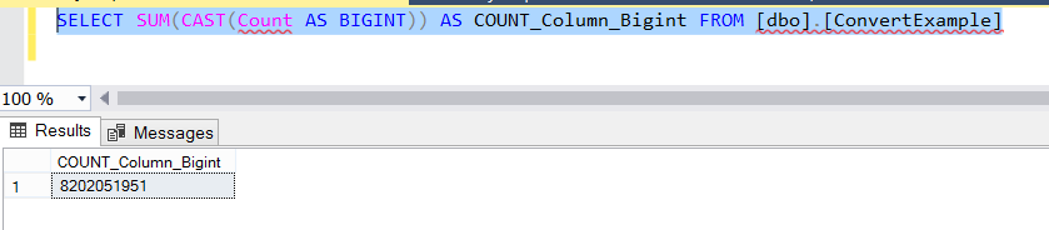Sunday , September 24 2023

# “Arithmetic overflow error converting expression to data type int.” Error When Using SUM Function

You can receive this error when you want to get a total result by using the SUM function. In order to avoid this error, you must convert the column in the SUM function to bigint type using CAST. Let’s clarify the subject by making an example.

Let’s create a table that has Name and Count columns and add a few records as follows. As you can see in the Script, the values we add to the Count column force the limits of the int data type.

When we sum count column with a query such as the following by using the SUM function, we will receive the error that is subject to our article because the total value will exceed the limit of the int type.When we change the query as follows, you will see that we can get the sum we want without error.